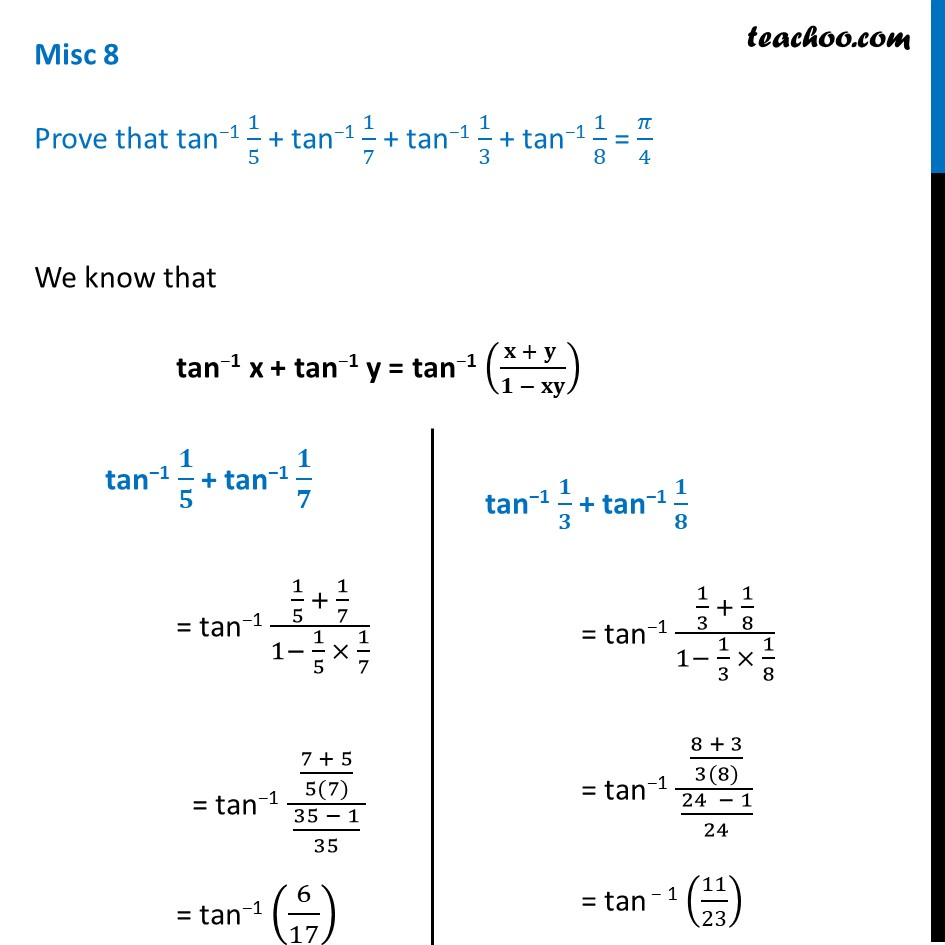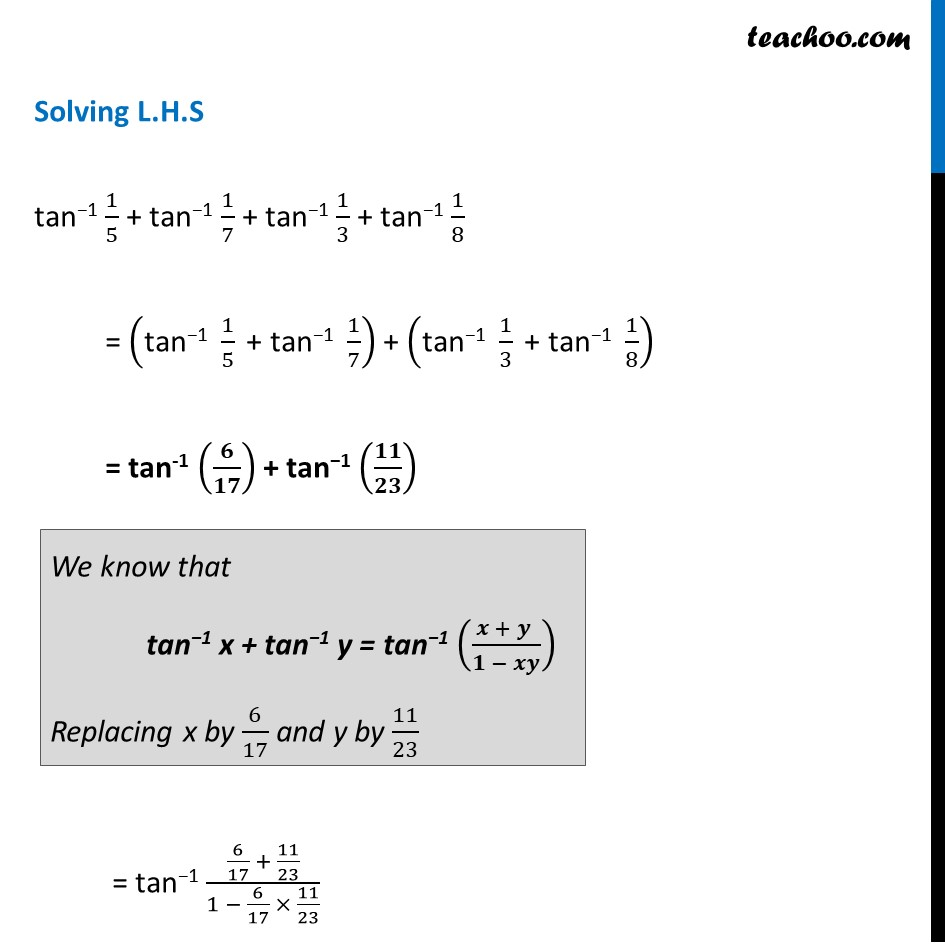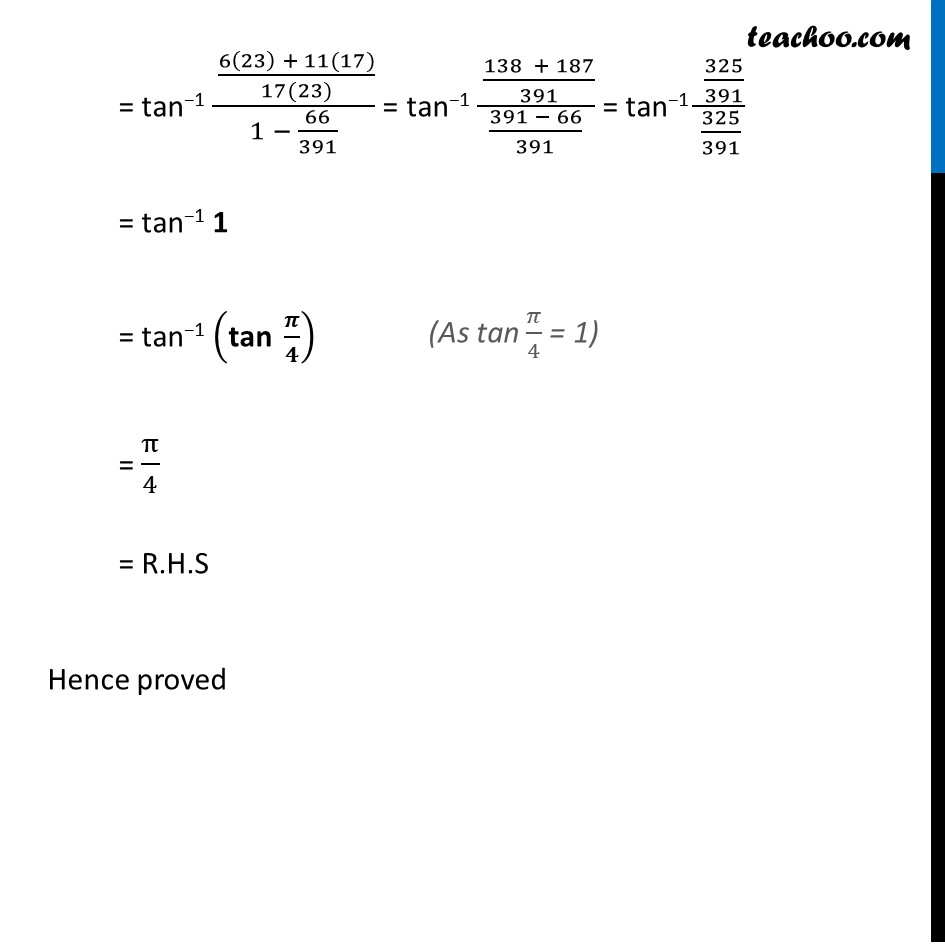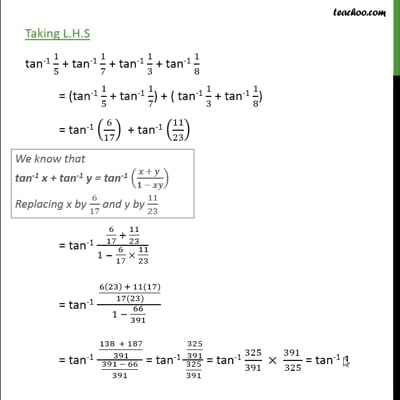Miscellaneous

Chapter 2 Class 12 Inverse Trigonometric Functions
Serial order wiseThis video is only available for Teachoo black users

Solve all your doubts with Teachoo Black (new monthly pack available now!)

### Transcript

Misc 8 Prove that tan−1 1/5 + tan−1 1/7 + tan−1 1/3 + tan−1 1/8 = 𝜋/4 We know that tan−1 x + tan−1 y = tan−1 ((𝐱 + 𝐲 )/(𝟏 − 𝐱𝐲)) tan−1 𝟏/𝟓 + tan−1 𝟏/𝟕 = tan−1 (1/5 + 1/7)/(1− 1/5 × 1/7) = tan−1 ((7 + 5)/(5(7)))/( (35 − 1)/35 ) = tan−1 (6/17) tan−1 𝟏/𝟑 + tan−1 𝟏/𝟖 = tan−1 (1/3 + 1/8)/(1− 1/3 × 1/8) = tan−1 ( (8 + 3)/(3(8)))/( (24 − 1)/24) = tan − 1 (11/23) Solving L.H.S tan−1 1/5 + tan−1 1/7 + tan−1 1/3 + tan−1 1/8 = ("tan−1 " 1/5 " + tan−1 " 1/7) + ("tan−1 " 1/3 " + tan−1 " 1/8) = tan-1 (𝟔/𝟏𝟕) + tan−1 (𝟏𝟏/𝟐𝟑) = tan−1 (6/17 + 11/23)/(1 − 6/17 × 11/23) We know that tan−1 x + tan−1 y = tan−1 ((𝒙 + 𝒚 )/(𝟏 − 𝒙𝒚)) Replacing x by 6/17 and y by 11/23 = tan−1 ( (6(23) + 11(17))/(17(23)))/(1 − 66/391) = tan−1 ( (138 + 187)/391)/((391 − 66)/391) = tan−1 ( 325/391)/(325/391) = tan−1 1 = tan−1 ("tan " 𝝅/𝟒) = π/4 = R.H.S Hence proved (As tan 𝜋/4 = 1)